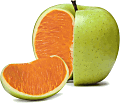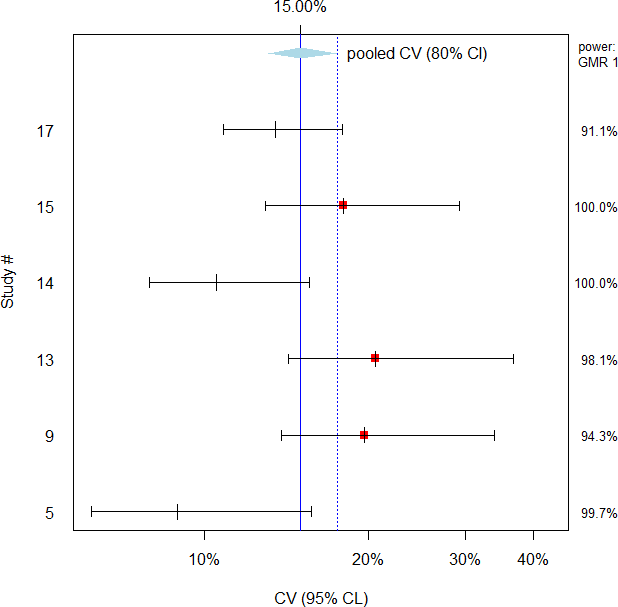## Pooling is fooling? [🇷 for BE/BA]

Hi ElMaestro and all,

❝ I will take it in good spirit that for some it can be difficult to accept diverging opinions without lashing out in a personal fashion. My ambition with my everyday dialogue is to keep the conversation on the healthy side of the fine line that separates humor from venomous hints.

If I crossed the line, please accept my sincere apologies.

❝ There is no particular scientific reason to assume variance homogeneity for studies done at different times, at different locations, under different designs, with different SOPs, using different equipment and protocols etc. If I am not mistaken this is what we are doing when we pool CVs like described here.

I examined data (CVintra of Cmax of MR MPH) from the public domain and some of my studies. Doesn’t matter whether a chiral method is used or not since the in vivo interconversion is negligible.
library(PowerTOST) CVs <- (" CV    |n |design|no|source                |type     |food    |reg|assay   |method 0.1971|33|3x6x3 |1 |Modi et al. 2000      |dose prop|fast    |SD |LC-MS/MS|chiral 0.2180|24|4x4   |2 |Midha et al 2001      |food eff |fast/fed|SD |GC/ECD  |chiral 0.1658|19|2x2x2 |3 |Markowitz et al. 2003 |BE       |fast    |SD |LC-MS/MS|achiral 0.1378|23|3x3   |4 |Rochdi et al. 2005    |dose prop|fast    |SD |LC-MS/MS|achiral 0.0890|12|2x2x2 |5 |Fischer et al. 2006   |sprinkle |fed     |SD |GC/MS   |achiral 0.2028|19|3x3   |6 |Patrick et al. 2007   |alcohol  |fed     |SD |LC-MS/MS|chiral 0.0870|24|3x3   |7 |Tuerck et al. 2007    |line ext |fast    |SD |LC-MS/MS|chiral 0.1415|27|4x4   |8a|Haessler et al. 2008  |BE       |fast    |SD |LC-MS/MS|achiral 0.1741|26|4x4   |8b|Haessler et al. 2008  |BE       |fed     |SD |LC-MS/MS|achiral 0.1965|13|2x2x2 |9 |Schütz et al. 2009    |BE       |fed     |SD |GC/MS   |achiral 0.1398|16|4x4   |10|Wang et al. 2004      |BE       |fast    |SD |LC-MS/MS|achiral 0.1202|12|4x4   |11|6520-9973-03          |food eff |fast/fed|SD |GC/MS   |achiral 0.2381|12|2x2x2 |12|6520-9979-04          |MR/IR    |fed     |SD |GC/MS   |achiral 0.2052|12|2x2x2 |13|EudraCT 2005-004375-38|BE       |fed     |SD |GC/MS   |achiral 0.1049|11|3x6x3 |14|EudraCT 2009-013059-31|pilot    |fed     |SD |GC/MS   |achiral 0.1793|15|2x2x2 |15|EudraCT 2009-015822-12|line ext |fed     |SD |GC/MS   |chiral 0.0854|16|2x2x2 |16|EudraCT 2010-021272-28|line ext |fed     |MD |GC/MS   |chiral 0.1347|18|3x6x3 |17|EudraCT 2011-002358-30|pilot    |fed     |SD |GC/MS   |chiral") txtcon <- textConnection(CVs) CVdata <- read.table(txtcon, header=TRUE, sep="|", strip.white=TRUE, as.is=TRUE) close(txtcon) alpha   <- 0.05 alphaCL <- 0.2 CVp   <- CVpooled(CVdata, alpha=alphaCL) for (j in seq_along(row.names(CVdata))) {   n <- CVdata$n[j] CVdata$pwr.GMR1[j] <- suppressMessages(power.TOST(CV=CVdata$CV[j], theta0=1, n=n, design=CVdata$design[j]))   CVdata$df[j] <- eval(parse(text= known.designs()[which(known.designs()[["design"]] == CVdata$design[j]), "df"], srcfile=NULL))   CL <- CVCL(CV=CVdata$CV[j], df=CVdata$df[j], side="2-sided", alpha=alpha)   CVdata$CLlo[j] <- signif(CL[["lower CL"]], 4) CVdata$CLhi[j] <- signif(CL[["upper CL"]], 4)   ifelse (CVdata$CLhi[j] > CVp$CVupper, CVdata$sig[j] <- "*", CVdata$sig[j] <- "ns")   CVdata$N.CV[j] <- sampleN.TOST(CV=CVdata$CV[j], design="2x2x2",                                     print=FALSE)[["Sample size"]]   CVdata$N.CL[j] <- sampleN.TOST(CV=CVCL(CV=CVdata$CV[j], df=CVdata$df[j], side="2-sided", alpha=alphaCL)[["upper CL"]], design="2x2x2", print=FALSE)[["Sample size"]] } CVdata$w.var <- CV2mse(CVdata$CV)*CVdata$df dftot        <- sum(CVdata$df) CVCL <- CVCL(CV=CVp$CV, df=dftot, side="2-sided", alpha=alpha) print(CVp, verbose=TRUE); print(CVdata, row.names=FALSE) ylim  <- c(1, max(as.numeric(row.names(CVdata)))+1) xlim  <- range(c(CVdata$CLlo, CVdata$CLhi)) xlab  <- sprintf("CV (%i%% CL)", 100*(1-alpha)) ylab  <- "Study #" ycorr <- 18*0.025/(diff(ylim)) dev.new(record=TRUE) op <- par(ask=TRUE) par(pty="s") plot(CVdata$CV, row.names(CVdata), type="n", log="x", axes=FALSE, frame.plot=TRUE, xlim=xlim, ylim=ylim, xlab=xlab, ylab=ylab) axis(1, at=pretty(xlim), labels=sprintf("%.0f%%", pretty(100*xlim))) axis(2, at=1:nrow(CVdata), labels=CVdata$no, tick=FALSE, las=1) axis(3, at=CVp$CV, labels=sprintf("%.2f%%", 100*CVp$CV)) abline(v=c(CVp$CV, CVCL[["upper CL"]]), lty=c(1, 3), col="blue") for (j in seq_along(row.names(CVdata))) { if (CVdata$CV[j] > CVCL[["upper CL"]])     points(CVdata$CV[j], j, pch=15, cex=1.1, col="red") arrows(x0=CVdata$CLlo[j], y0=j, x1=CVdata$CLhi[j], y1=j, length=ycorr*2, angle=90, code=3) points(CVdata$CV[j], j, pch=3, cex=1.5)   mtext(4, text=sprintf("%4.1f%%", 100*CVdata$pwr.GMR1[j]), at=j, line=2.6, las=1, cex=0.85, adj=1) } loc <- max(as.numeric(row.names(CVdata)))+1 polygon(x=c(CVCL[["lower CL"]], CVp$CV, CVCL[["upper CL"]],             CVp$CV, CVCL[["lower CL"]]), y=c(loc, loc-ycorr*8, loc, loc+ycorr*8, loc), border=NA, col="lightblue") text(x=CVCL[["upper CL"]], y=loc, pos=4, labels=paste0("pooled CV (", 100*(1-alphaCL), "% CI)")) mtext(4, text="power:\nGMR 1", at=loc, line=0.5, las=1, cex=0.85) CVset1 <- subset(CVdata, no %in% c("5", "9", "13", "14", "15", "17")) CVp1 <- CVpooled(CVset1, alpha=alpha) for (j in seq_along(row.names(CVset1))) { ifelse (CVset1$CV[j] > CVp1$CVupper, CVset1$sig[j] <- "*",                                        CVset1$sig[j] <- "ns") } CVset1$w.var <- CV2mse(CVset1$CV)*CVset1$df dftot        <- sum(CVset1$df) CVCL <- CVCL(CV=CVp1$CV, df=dftot, side="2-sided", alpha=alpha) print(CVp1, verbose=TRUE); print(CVset1, row.names=FALSE) CVset1$study <- seq_along(1:length(CVset1$n)) ylim  <- c(1, nrow(CVset1)+1) ycorr <- 18*0.025/(diff(ylim)) plot(CVset1$CV, 1:nrow(CVset1), type="n", log="x", axes=FALSE, frame.plot=TRUE, xlim=xlim, ylim=ylim, xlab=xlab, ylab=ylab) axis(1, at=pretty(xlim), labels=sprintf("%.0f%%", pretty(100*xlim))) axis(2, at=1:nrow(CVset1), labels=CVset1$no, tick=FALSE, las=1) axis(3, at=CVp1$CV, labels=sprintf("%.2f%%", 100*CVp1$CV)) abline(v=c(CVp1$CV, CVCL[["upper CL"]]), lty=c(1, 3), col="blue") for (j in seq_along(row.names(CVset1))) { if (CVset1$CV[j] > CVCL[["upper CL"]])     points(CVset1$CV[j], j, pch=15, cex=1.1, col="red") arrows(x0=CVset1$CLlo[j], y0=j, x1=CVset1$CLhi[j], y1=j, length=ycorr/1.5, angle=90, code=3) points(CVset1$CV[j], j, pch=3, cex=1.5)   mtext(4, text=sprintf("%4.1f%%", 100*CVdata$pwr.GMR1[j]), at=j, line=2.6, las=1, cex=0.85, adj=1) } loc <- nrow(CVset1)+1 polygon(x=c(CVCL[["lower CL"]], CVp1$CV, CVCL[["upper CL"]],             CVp1\$CV, CVCL[["lower CL"]]),         y=c(loc, loc-ycorr, loc, loc+ycorr, loc),         border=NA, col="lightblue") text(x=CVCL[["upper CL"]], y=loc, pos=4,      labels=paste0("pooled CV (", 100*(1-alphaCL), "% CI)")) mtext(4, text="power:\nGMR 1", at=loc, line=0.5, las=1, cex=0.85) par(op)

and got this ( denote CVs > the upper CL of the pooled CV; power for a GMR of 1 in the right margin):Oops! Apples and oranges.
What we also see: Variability was the highest in study #12 (MR vs. IR), whereas MD (#16) showed only half of the variability of SD (#15) although the accumulation was <1%.

For the subset (same product, SD, same analytical method):Here using the upper CL of the pooled CV would “work” but using the highest CV would be even more conservative.

Dif-tor heh smusma 🖖🏼 Довге життя Україна!Helmut SchützThe quality of responses received is directly proportional to the quality of the question asked. 🚮
Science QuotesIng. Helmut Schütz